RUSSIAN JOURNAL OF EARTH SCIENCES, VOL. 20, ES2003, doi:10.2205/2020ES000707, 2020

# Methodology of physiography zoning using machine learning: A case study of the Black Sea

Denis Krivoguz

"Fisheries Oceanography" department, Research Institute of the Azov Sea Fishery Problems (AzNIIRKH), Kerch, Russia

### Abstract

Problem of area's zoning is very important and is one of the main problems of modern geographical science. Our point is to from a modern approach, based on the machine learning methods to provide zoning of any area. Key ideas of this methodology, that any distribution of factors that form any geographical system grouped around some clusters – unique zones that represents specific nature conditions. Formed methodology based on several stages – selection of data and objects for analysis, data normalization, assessment of predisposition of data for clustering, choosing the optimal number of clusters, clustering and validation of results. As an example, we tried to zone a surface layer of the Black Sea. We find that optimal number of unique zones is 3. Also, we find that the key driver of zone forming is a location of the rivers. Thus, we can say, that applying a machine learning approach in area's zoning tasks helps us increasing the quality of nature using and decision-making processes.

### 1. Introduction

The problem of zoning has always been and will be the main problem of geographical science. In this context, region or zone is the main territorial system, which is always part of larger regional units. Based on this, zoning is the process of identifying and studying the objectively existing territorial structure, organization, and hierarchical subordination of physical and geographical complexes. Zoning of any area includes several important goals [Vinokurov et al., 2005; Zaika 2014]:

1. Finding an existing physiography complexes;
2. mapping of physiography maps;
3. deep understanding of the complex composition;
4. research of processes and factors, that are forming complexes;
5. complex classification;
6. Finding of any interactions between factors or complexes;
7. developing of physiography zoning methods.

Thus, the main goal of this paper was to form a modern mathematical methodology, based on machine learning methods to provide zoning of any area.

In the last years problem of area's zoning and its methodology was tried to solve by several authors.

For example Skrebets and Pavlova  conducted a physical and geographical zoning of the Black Sea using correlation analysis. They used a mapping based on relationship between phytoplankton and natural factors, that limiting its distribution. Using this approach, they identified 5 regions that differ from each other in quantitative way, as well as in combination of relationships.

From a biological point of view, this problem was considered by

Zaika . He carried out biological zonation of the Black Sea and also described the main problems of its implementation. The principle of distinguishing different regions was based on quantitative analysis of the dominant species in different regions of the Black Sea.

The widespread use of physiographic zonation received in landscape ecology. Vinokurov et al.  proposed a methodology and implemented the physical and geographical zoning of Siberia. Based on various natural features, they identified more than 100 different regions with unique physical and geographical conditions.

Tamaychuk  in his paper tried analytical approach to zoning Black Sea area, based on main factors of spatial differentiation, distribution features of environmentally significant characteristics and modern ideas about the theory and methods of physiographic zoning. He divided area of the Black Sea into 3 water-provinces – North-West moderate, North-East moderate and subtropical.

Mathematical approach was shown in Sovga et al.  work. They used depth, mean values of temperature and salinity, differences and features in flora and fauna as a factor. They divided area of the North-West part of the Black Sea into 4 groups – West, Karkinitsky, Central and Kalamitsky.

V. Agostini [Agostini et al., 2015] in her paper tried to make a zoning of marine environment in St. Kitts and Nevis. For her analysis, she used 37 spatial layers, that represent different factors and fully described functionality of the research area, that was divided into 3 major groups – "habitat", "species" and "human use". As the result, she distinguished 4 major zones – "conservation", "transportation", "touristic" and "fishing".

Petrov and Bobkov  tried to form the concept of hierarchical structure of large marine ecosystems in the Arctic shelf of Russia. Based on environmental variables, they distinguished 7 eco-regions of the Barents Sea – South-Western, Pechora Sea, Central basin south, Central basin north, Novaya Zemlya shore, Svalbard Archipelago and Franz Josef Land Archipelago.

Fyhr et al.  tried to review all of the modern concepts and tools for Ocean zoning. Based on their work, the most actual and commonly used tools are Atlantis, Cumulative Impacts Assessment Tool, Integrated Valuation of Ecosystem Services and Tradeoffs (InVEST), Marine Protected Areas Decision Support Tool (Marine Map), Marxan and Marxan with Zones, NatureServe Vista and Zonation.

### 2. Clustering as Physiographic Zoning Method

Clustering is a task of dividing the entire dataset into separate groups of homogenous objects, that are similar to each other, but have distinct difference between this separate groups [Aleshin and Malygin, 2019]. Clustering algorithms are divided in two groups – hierarchical and iterative.

I. Hierarchical – consistently build clusters from already found clusters.

1. Agglomerative (unifying) – start with individual elements, and then combine them;
2. separation – start with one cluster, and then – divide them;

II. Non-hierarchical – optimize a certain objective function.

1. Graph theory algorithms;
2. EM algorithm;
3. $K$-means algorithm ($k$-means clustering);
4. fuzzy algorithms.

Any clustering algorithm can be considered effective if the compactness hypothesis is satisfied [Shi and Horvath, 2006].

Physiographic zoning using clustering method is carried out in several stages:

1. Selection of data and objects for analysis;
2. data normalization;
3. assessment of predisposition of data for clustering;
4. choosing the optimal number of clusters;
5. clustering and validation of results.

Formally, almost all clustering tasks come down to this form. Let $X$ be the set of objects, $Y$ is the set of numbers (names, labels) of clusters. The distance function between objects is specified as $\rho(x,x\prime)$ [Collins et al., 2002]. There is a finite training set of objects $X^m={x_1,...,x_n}\in X$. So, the main goal of clustering is to divide dataset into several disjoint subsets. These subsets called clusters and consist from objects, that are closed to the $\rho$-metric. Objects from different clusters were significantly different. For every object $x_i\in X^m$ assigned the number of cluster $y_i$ [Marron et al., 2014].

#### 2.1. Data Normalization

Data normalization is one of the feature transformation operations that is performed during their generation at the data preparation stage. In case of machine learning, normalization is a procedure for preprocessing input information (training, test and validation samples, as well as real data), in which the values of the attributes in the input vector are reduced to a certain specified range of values, for example: $[0...1]$ or $[-1...1]$.

The importance of data normalization comes from the nature of algorithms and models in machine learning. The values of raw data can vary in a very wide range and differ from each other by several orders [Rybkina et al., 2018]. The work of such machine learning models like neural networks or Kohonen self-organizing maps with not normalized data will be incorrect – difference between attribute's values can cause instability of the model, that will lead to worth learning results and slowing the modelling process. Also, some parametric machine learning models require symmetric and unimodal data distribution. After normalization, all the numerical values of the input attributes will be reduced to the same amount – a certain narrow range [Criminisi et al., 2012].

There are many ways to normalize feature values in order to scale them to a single range and use them in various machine learning models. Depending on the function used, they can be divided into two large groups: linear and non-linear [Tealab et al., 2017]. With nonlinear normalization, the calculated ratios use the functions of the logistic sigmoid or hyperbolic tangent. In linear normalization, the change of variables is carried out proportionally, according to a linear law.

The most common methods for data normalization are:

Minimax – linear data transformation in the range $[0..1]$, where the minimum and maximum scalable values correspond to 0 and 1, respectively:

\begin{eqnarray*} X_{\mathrm{norm}}=\frac{X-X_{\min}}{X_{\max}-X_{\min}} \end{eqnarray*}

$Z$-scaling based on the mean and standard deviation: dividing the difference between the variable and the it means by the standard deviation:

\begin{eqnarray*} z=\frac{x-\mu}{\sigma} \end{eqnarray*}

Decimal scaling – performed by removing the decimal separator of the variable value [Seber and Lee, 2003].

In practice, minimax and $Z$-scaling have similar areas of applicability and are often interchangeable. However, in calculating the distances between points or vectors in most cases, $Z$-scaling is used, while minimax is useful for visualization.

#### 2.2. Assessment of Predisposition of Data for Clustering

One of the most common problem of unsupervised machine learning is that clustering will form groups, even if the analyzed dataset is a completely random structure. That's why the first validation task that should be applied even before clustering is to assess the overall predisposition of the available data to cluster tendency [Sivogolovko and Thalheim, 2013].

There are two common indicators, that can show us cluster tendency – Hopkins statistics and Visual Assessment of cluster Tendency or "VAT diagram".

To calculate Hopkins statistics, we need to create B pseudo-datasets, randomly generated based on the distribution with the same standard deviation as the original dataset. For each observation $i$ from $n$, the average distance to $k$ nearest neighbors is calculated as follows: $w_i$ between real observations and $q_i$ between generated observations and their closest real neighbors [Keller et al., 1985; Sivogolovko and Thalheim, 2013]. Then the Hopkins statistics calculates as follows:

\begin{eqnarray*} H_{\mathrm{ind}} = H_{\mathrm{ind}}=\frac{\sum_{n}w_i}{\sum_{n}q_i+\sum_{n}w_i} \end{eqnarray*}

If $H_{\mathrm{ind}}>0.5$, then it will correspond to the null hypothesis that $q_i$ and $w_i$ are similar and values are distributed randomly and uniformly. If $H_{\mathrm{ind}} < 0.25$ this indicates that a dataset has a tendency to data grouping.

For visual assessment of clustering tendency, the best way is to using VAT diagram. VAT algorithm consists of:

1. Compute the dissimilarity matrix between the objects in the data set using the Euclidean distance measure;
2. reorder the dissimilarity matrix so that similar objects are close to one another. This process creates an ordered dissimilarity matrix;
3. the ordered dissimilarity matrix is displayed as an ordered dissimilarity image, which is the visual output of VAT.

The VAT detects the clustering tendency in a visual form by counting the number of square shaped dark blocks along the diagonal in a VAT image [Sivogolovko and Thalheim, 2013].

#### 2.3. Choosing the Optimal Number of Clusters

At this moment there's two main ways to choose an optimal number of clusters – "elbow" method and using of gap statistics [Chapelle et al., 2006].

The "elbow" method – considered the pattern of variation in the dispersion of $W_{\mathrm{total}}$ with increasing in number of groups $k$ [Tomar et al., 2018]. Combining all of the founded observations in one group, we'll have the biggest intraclass dispersion, that will decrease to 0 when $k\rightarrow n$. The point, when this decreasing of dispersion will be slowing down, called "elbow" [Seber and Lee, 2003; Thiery et al., 2006].

An alternative to the "elbow" method is using gap statistics, which are generated based on resampling and Monte-Carlo simulation processes. For example, let $E_n^\ast{\log(W_k^\ast)}$ denotes the valuation of average dispersion $W_k^\ast$, obtained by bootstrap method, when $k$ clusters are formed by several random objects $f$ from the original dataset of $n$ size. Then gap statistics will be calculated as follows:

\begin{eqnarray*} \mathrm{Gap}_n(k)=E_n^\ast{\log(W_k^\ast)}-\log(W_k) \end{eqnarray*}

$\mathrm{Gap}_n(k)$ determines the deviation of the observed dispersion $W_n$ from its expected value, if the original data formed only one cluster.

#### 2.4. Validation of Clustering Results

Currently, there are several ways to validate the results of clustering:

1. External validation – comparing the results of cluster analysis with already known validation dataset;
2. relative validation – evaluating the structure of formed clusters by changing the algorithm parameters;
3. internal validation – obtaining internal information of clustering process;
4. assessment of the clustering stability using resampling.

The most widespread indexes are silhouette index and Calinski-Harabasz index [Sivogolovko and Thalheim, 2013].

One of the approaches to validate the results of clustering is the Calinski-Harabasz index.

Let ${\overline{d}}^2$ is the mean square distance between elements in clustering variety and ${\overline{d}}_{c_i}^2$ – mean square distance between elements in cluster $c_i$. Then the distance inside groups will be:

\begin{eqnarray*} \mathrm{WGSS} = \frac{1}{2}\sum_{i=1}^{c}(n_{c_i}-1){\overline{d}}_{c_i}^2 \end{eqnarray*}

and the distance between groups will be:

\begin{eqnarray*} \mathrm{BGSS} = \frac{1}{2}\left(\left(c-1\right) {\overline{d}}^2+\left(N-c\right)A_c\right) \end{eqnarray*}

where $a_c = A_c/\overline{d}^2$ – is weighted mean difference of distances between cluster centers and a mutual variety center. Then the Calinski-Harabasz index will be:

\begin{eqnarray*} \mathrm{VRC} = \frac{\mathrm{BGSS}/(c-1)}{\mathrm{WGSS}/(N-c)} = \end{eqnarray*} \begin{eqnarray*} \frac{{\overline{d}}^2+ [(N-c)/(c-1)]A_c}{{\overline{d}}^2-A_c} = \end{eqnarray*} \begin{eqnarray*} \frac{1+[(N-c)/(c-1)]a_c}{1-a_c} \end{eqnarray*}

where $a_c=A_c/\overline{d}^2$. We can see, that if the all distances between points are similar, then $a_c=0$ and $\mathrm{VRC} = 1$. $a_c=1$ characterize the prefect clustering. The maximum value of corresponds to optimal cluster's structure.

Another approach to validate the clustering results is using the silhouette index. Its values shows the degree of similarity between object and cluster that he belongs to, compared to another clusters [Shi and Horvath, 2006; Soliman et al., 2017].

Silhouette of every cluster estimates as follows: let object $x_j$ corresponds to cluster $c_p$. Denote the mean distance from this object to other objects from this cluster $c_p$ as $a_{pj}$ and the mean distance from this object $x_j$ to objects from another cluster as $c_q,q \neq p$ as $d_{q,j}$. Let $b_{pj} = \min_{q\neq p}d_{qj}$. This value means the measure of dissimilarity of single object with objects from nearest cluster. Thus, the silhouette of every single element of cluster calculates as:

\begin{eqnarray*} S_{x_j}=\frac{b_{pj}-a_{pj}}{\max(a_{pj},b_{pj})} \end{eqnarray*}

The highest values of $S_{x_j}$ corresponds to better affiliation of element $x_j$ to cluster $p$. The evaluation of all cluster structure provided by averaging the value by elements:

\begin{eqnarray*} \mathrm{SWC} = \frac{1}{N}\sum_{j=1}^{N}S_{x_j} \end{eqnarray*}

Better clustering characterized by bigger values of , that achieved when the distance inside cluster $a_{pj}$ is small and the distance between objects from neighboring clusters $b_{pj}$ is big.

### 3. Black Sea Surface Physiographic Zoning

#### 3.1. Research Area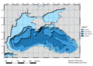Figure 1

The Black Sea is an inland sea, that belongs to the basin of the Atlantic Ocean. Its maximum depth reaches the mark of 2258 meters (Figure 1) [Barratt, 1993]. The total area of the Black Sea is 420,325 km$^2$, and with the Sea of Azov – 462,000 km$^2$ [Murray, 2005].

The average seasonal cycle of geostrophic circulation of the Black Sea [Ivanov and Belokopytov, 2011]:

• From January to March – a single cyclonic rotation with a center in the eastern part of the sea, the western circulation is weakly expressed;
• from April to May – a single cyclonic rotation with a center in the western part of the sea, the eastern cycle is weakly expressed;
• from June to July – two cycles, the western more intense;
• from August to September – two cycles, the eastern one is more intense;
• from October to December – two cycles of equal intensity.

About 80% of the river flow is concentrated in the northwestern part of the Black Sea. The Caucasian rivers contribute about 13% of the water balance, while the runoff from Turkeys rivers is about 7% [Ghervas 2017]. The contribution of the Crimean rivers a is insignificant [Belokopytov and Shokurova, 2005].

The biggest river, that flows into the Black Sea is Danube. The Danube usually brings about 203 km$^3$ of freshwater into North-Western part of the Black Sea, decreasing the level of salinity there. Another big river, that flows into Black Sea is Dnieper from Ukrainian part and Rioni from Georgian [Ozsoy and Unluata, 1997].

#### 3.2. Data

We used the monthly averaged data from Copernicus Marine Environmental Monitoring Service (CMEMS) – Black Sea Reanalysis, which are based on 5 components:

1. Ocean model – Hydrodynamic model, which is a part of the NEMO (Nucleus for European Modelling of the Ocean) project;
2. scheme of data assimilation (OceanVar) for temperature and salinity profiles, satellite data for sea surface temperature, sea level anomalies etc.;
3. assimilated data – in-situ data for environmental variables;
4. recovery scheme for environmental variables;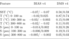Table 1

Data from this model have a high level of correlation with in-situ data, that increasing with depth. For example, the accuracy of temperatures spatial distribution in the Black Sea at depth of 30 m about $\pm{1.5}$° C, at the depth of 70 m it decreases to $\pm{0.3}$° C and at the depth of 1100 m is about $\pm{0.04}$° C (Table 1).

The quality of the model data, as well as the model itself, improve with increasing of in-situ observations numbers.

For Black Sea surface physiographic zoning we used 6 environmental parameters – sea surface temperature, sea surface salinity, dissolved oxygen level, PO$_4$ and NO$_3$ content and primary production level.

#### 3.3. Results

To understand, does dataset has a tendency to form clusters, we calculated a Hopkins index using the R-package "clustertend". It was equal to 0.0194, that means that this dataset can form clusters.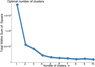Figure 2

To estimate an optimal number of clusters, we used the R-package "factoextra". Results shown in Figure 2.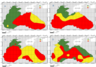Figure 3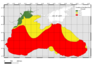Figure 4

As we can see at the Figure 2, the elbow of our curve is located at 3, thus we can distinguish 3 completely different zones in the surface waters of the Black Sea (Figure 3, Figure 4). Allocation of this zones due equally to all of analyzed factors, except dissolved oxygen.

Based on statistical analysis all of these factors divided in two groups. First – phosphates concentration, primary production and chlorophyll-$\alpha$, which are derivatives from each other – the amount of phosphates impacts on amount of primary production and amount of primary production impacts on amount of produced chlorophyll-$\alpha$. Second are temperature, salinity and nitrates concentration.

Studying water objects, it's important to know a seasonal variability of zones, because of its very high change capability in time. Comparing with land, water systems aren't stable for long period of time and spatial distribution of factors can vary from season to season.

Generally, as we can see in figure, main reasons of zoning pattern forming are quantitative and qualitative characteristics on flows.

In winter season, there is a clear divide of the Black Sea from west to east. A significant role in this process is played by the interaction of the Black Sea with the Sea of Marmara, river flows in the northwest of the Black Sea and in the Caucasus and, in some cases, areas near the Southern coast of Crimea and the Kerch Peninsula due to the activity of currents from the Sea of Azov.

In spring season, the divide of the Black Sea occurs from north to south. In this case, a significant impact on this process is exerted by the significant flow of such rivers as the Dniester, Danube and Dnieper in the north-west of the Black Sea and the influx of water from the Sea of Marmara. Due to the interaction between two water masses radically different in their characteristics, it forms an intermediate zone between them, covering an area from the Kerch Strait to the Danube Delta.

In the summer, due to the nature of the internal currents in the Black Sea and changes in the volume of river flow, more saline water from the Sea of Marmara reaches the Danube. In spatial terms, the pattern of zones distribution in the Black Sea is similar to the winter one, in which they are located from east to west. The formation of the intermediate second zone is most likely due to the interaction with more fresh and cold water coming from the Sea of Azov.

In autumn, the formation of more fresh and colder waters off the coast of Turkey is observed, which is due to the significant flow of the rivers of the Turkish coast. The distribution pattern is more similar to the spring one, with significantly increased in size zone 1.

Annual zoning of the Black Sea is presented on figref{4}.

Zone 1. Located in the North-West part of the Black Sea. Flows from Danube, Dniester, Dnieper and Southern Bug completely equal of 3/4 of a total flow into the Black Sea. Dominated northern and north-western winds helps in spreading of matters, endured by rivers. The main feature of this part of the sea is an active interaction of fresh water from rivers with salty water from south of the Black Sea. Near the shore water salinity reaches values about $7-8 \pm$. Temperature of water surface, as a salinity, increasing from shore to open sea. Temperature differences reaches 1.5–2.0° C. Bioproductivity of this zone is quite high, mainly cause of active flowing rivers matter and fresh water. But local hydrophysical and hydrochemical conditions condition high variability of bioproductivity with fishkills.

Zone 2. Basically, forming of this zone determined by interactions between 1-st and 3-rd zones, where as a results of Black Sea currents and flows from big rivers, cold fresh water from the coastal areas mixed up with more cold and salty water from central part of the Black Sea. Located in the north-west part of the Black Sea, near the Crimean-Caucasus shore of Russia, Georgian and Turkey coasts. Biggest rivers here are Rioni, Tuapse, Kizilirmak, Yesilirmak and Inguri. Like the zone 1, location of the zone 2 is due to the flows from rivers. But cause of lower levels of flow amount, compared with the zone 1, their impact on water of the Black Sea is quite lower, but noticeable. Values of salinity here doesn't differ from the central part ($1-2 \pm$ fresher), same as a temperature.

Zone 3. Natural conditions of this zone are a common to the Black Sea. The area of this zone is the biggest. Located in the south and central part of the Black Sea and near the Kerch Strait. Salinity here is a quite high – $19-20 \pm$, and reaches $24 \pm$ near the Bosporus Strait. The impact of the Sea of Azov is quite low, due to specificity of Azov currents. Amount of phosphates and nitrates is low due to lack of any big rivers, which are the main sources of their presence in the sea water. As a result, concentrations of chlorophyll-$\alpha$ is quite low too.

### 4. Conclusions

Thus, the methodological approach, showed in this paper, helps us to use it fully in zoning tasks to provide distinguishing from them completely different areas, that aren't similar. As we can see, the main advantages of this approach are lack of subjectivity that is inherent to humans, high level of analysis accuracy, possibility of constant model's modification by adding new in-situ data or by modifying the algorithm itself. Also, it should be noted, that the indisputable advantage of this approach is the ability to use it in any kind of territory, both in size and in properties.

As we talk about disadvantages of this approach, we should note a strong dependency from input data quality and data normalization, which in some cases can lead to significant distortion in the analysis results. The same we can say about data size. With significant amount of data, it may be difficult to conduct the research, which leads to completely change the used algorithm or to significant reduction in data size and, as a result, to simplification of the model and distortion of the real results. Generally, we should note, that using of this approach is justified in most cases, but the need of improvement and further optimization of it doesn't disappear.

Obtained results helps us to understand that applying of this approach can helps us to go away from analytical and empirical zoning approaches to have a math basis, uniformity of calculations and process automatization. Conducted as an example of this approach application, Black Sea physiographic zoning generally is quite similar with previous works. It was determined, that the most optimal number of the dissimilar groups, based on analyzed factors is 3. Generally, their spatial location based on places where rivers flows into the Black Sea, and as a result more comfortable for different flora and fauna. For example, the conditions, that formed in the second area is quite comfortable for spawning of many commercial fishes, Like Liza haematocheilus, Engraulis encragicolus, Liza aurata, Mugil cephalus, etc. Thus, applying a machine learning approach in area's zoning tasks helps us to increase the quality of nature using and decision-making process.

### References

Agostini, V. N., S. W. Margles, et al. (2015) , Marine zoning in St. Kitts and Nevis: A design for sustainable management in the Caribbean, Ocean &amp; Coastal Management, 104, p. 1–10, https://doi.org/10.1016/j.ocecoaman.2014.11.003.

Aleshin, I. M., I. V. Malygin (2019) , Machine learning approach to inter-well radio wave survey data imaging, Russian Journal of Earth Sciences, 19, no. 3, p. ES3003, https://doi.org/10.2205/2019ES000664.

Barratt, L. (1993) , Black Sea oceanography, Reviews in Fish Biology and Fisheries, 3, no. 2, p. 199–200, https://doi.org/10.1007/bf00045240.

Belokopytov, V. N., I. G. Shokurova (2005) , Estimates of Temperature and Salinity Interdecadal Variability in the Black Sea in 1951–1995, Marine Hydrophysical Institute, Sevastopol.

Chapelle, O., B. Scholkopf, A. Zien (2006) , Semi-Supervised Learning, MIT Press, Massachusetts.

Collins, M., R. E. Schapire, Y. Singer (2002) , Logistic Regression, AdaBoost and Bregman Distances, Machine Learning, 48, no. 1/3, p. 253–285, https://doi.org/10.1023/A:1013912006537.

Criminisi, A. (2012) , Decision Forests: A Unified Framework for Classification, Regression, Density Estimation, Manifold Learning and Semi-Supervised Learning, Foundations and Trends in Computer Graphics and Vision, 7, no. 2–3, p. 81–227, https://doi.org/10.1561/0600000035.

Fyhr, F., A. Nillson, N. Sandman (2013) , A review of Ocean Zoning tools and Species distribution modelling methods for Marine Spatial Planning, Estonian Marine Institute, University of Tartu, Tallin.

Ghervas, S. (2017) , The Black Sea, Cambridge University Press, Cambridge.

Ivanov, V. A., V. N Belokopytov (2011) , Oceanography of the Black Sea, Marine Hydrophysical Institute, Sevastopol.

Keller, J. M., M. R. Gray, J. A. Givens (1985) , A fuzzy $K$-nearest neighbor algorithm, IEEE Transactions on Systems, Man, and Cybernetics, SMC-15, no. 4, p. 580–585, https://doi.org/10.1109/TSMC.1985.6313426.

Marron, D., A. Biffet, G. Morales (2014) , Random forests of very fast decision trees on GPU for mining evolving big data streams, Frontiers in Artificial Intelligence and Applications, ECAI 2014, p. 615–620, IOS Press, Amsterdam.

Murray, J. W. (2005) , Special Issue on Black Sea Oceanography, Oceanography, 18, no. 2, p. 14–15, https://doi.org/10.5670/oceanog.2005.37.

Ozsoy, E., U. Unluata (1997) , Oceanography of the Black Sea: a review of some recent results, Earth-Science Reviews, 42, p. 231–272, https://doi.org/10.1016/S0012-8252(97)81859-4.

Petrov, K. M., A. A Bobkov (2017) , The Concept of Hierarchical Structure of Large Marine Ecosystems in the Zoning of Russian Arctic Shelf Seas, The Interconnected Arctic – UArctic Congress 2016, Eds. K. Latola, H. Savela, Springer, Cham, Switzerland, https://doi.org/10.1007/978-3-319-57532-2_4.

Rybkina, A., S. Hodson, A. Gvishiani, P. Kabat, R. Krasnoperov, O. Samokhina, E. Firsova (2018) , CODATA and global challenges in data-driven science, Russian Journal of Earth Sciences, 18, no. 4, p. ES4002, https://doi.org/10.2205/2018ES000625.

Seber, G. A., A. J Lee (2003) , Linear Regression Analysis, Wiley-Interscience, New Jersey.

Shi, T., S. Horvath (2006) , Unsupervised Learning With Random Forest Predictors, Journal of Computational and Graphical Statistics, 15, no. 1, p. 118–138, https://doi.org/10.1198/106186006X94072.

Sivogolovko, E., B. Thalheim (2013) , Semantic Approach to Cluster Validity Notion, Advances in Databases and Information Systems, p. 615–620, IOS Press, Berlin, https://doi.org/10.1007/978-3-642-32741-4_21.

Skrebets, G. N., S. M. Pavlova (2019) , Physico-geographical zoning of ghe open waters of the Black Sea with help of correlation analysis, Proceedings of the V. I. Vernadsky Crimean Federal University, 5, no. 1, p. 87–96.

Soliman, A., K. Soltani, J. Yin, A. Padmanabhan, S. Wang (2017) , Social sensing of urban land use based on analysis of Twitter users' mobility patterns, PLOS ONE, 12, no. 7, p. e0181657, https://doi.org/10.1371/journal.pone.0181657.

Sovga, E. E., V. A. Zhorov, et al. (2005) , Zoning of the north-west part of the Black Sea according to mathematical modelling of the sore ecosystems, Ecological Safety of Coastal and Shelf Zones of Sea, 12, p. 421–428.

Tamaychuk, A. N. (2017) , The space heterogeneity of natural conditions and the division of the Black Sea, Ecological Safety of Coastal and Shelf Zones of Sea, 149, no. 2, p. 30–50.

Tealab, A., H. Hefny, A. Badr (2017) , Forecasting of nonlinear time series using ANN, Future Computing and Informatics Journal, 2, no. 1, p. 39–47, https://doi.org/10.1016/j.fcij.2017.05.001.

Thiery, Y., J. Malet, O. Maquaire (2006) , Test of fuzzy logic rules for landslide susceptibility assessment, Proceedings International Conference on Spatial Analysis and Geomatics, Strasbourg, France, CD-Rom Support Proceedings, p. 16p, SAGEO 2006, Strasbourg.

Tomar, P., R. Mishra, K. Sheoran (2018) , Prediction of quality using ANN based on Teaching-Learning Optimization in component-based software systems, Software: Practice and Experience, 48, no. 4, p. 896–910, https://doi.org/10.1002/spe.2562.

Vinokurov, Ju. I., et al. (2005) , Physiography zoning of the Siberia as a basis of regional natural-using system development, Polzunovsky Vestnik, 4, p. 3–13.

Zaika, V. E. (2014) , The problems of the Black Sea biotic regioning and conception of biotopes heterogeneity, Marine Ekological Journal, 8, no. 2, p. 5–13.

Received 19 February 2020; accepted 13 March 2020; published 22 March 2020.Citation: Krivoguz Denis (2020), Methodology of physiography zoning using machine learning: A case study of the Black Sea, Russ. J. Earth Sci., 20, ES2003, doi:10.2205/2020ES000707.

Generated from LaTeX source by ELXfinal, v.2.0 software package.